# A survey on methods and applications of meta-learning with GNNs

19. Apr 2021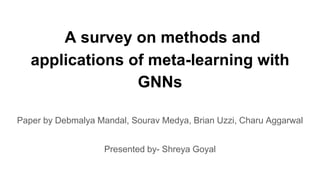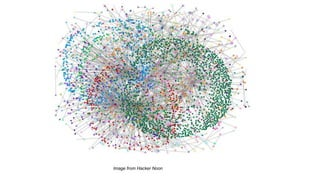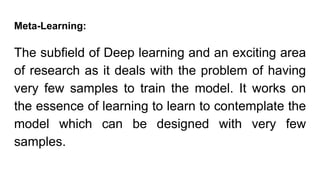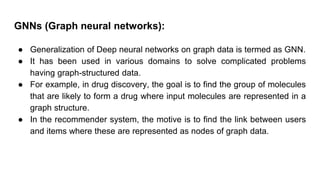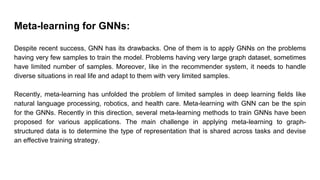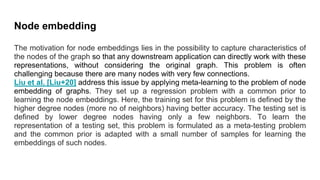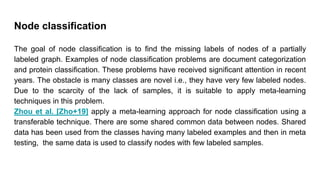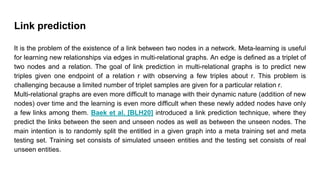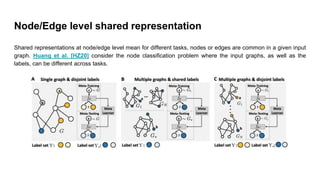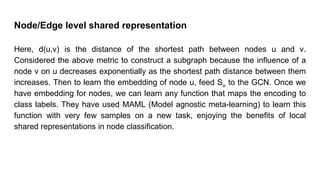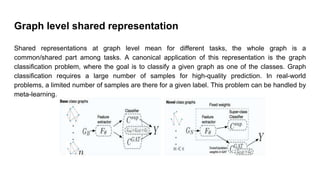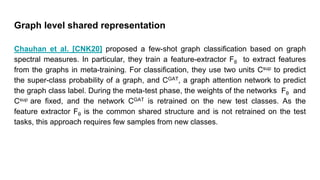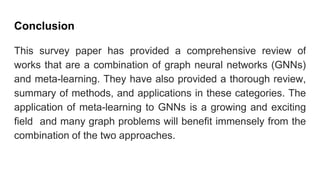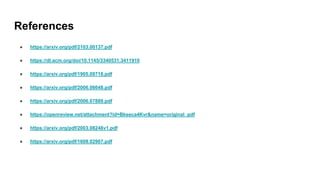1 von 14

### A survey on methods and applications of meta-learning with GNNs

• 1. A survey on methods and applications of meta-learning with GNNs Paper by Debmalya Mandal, Sourav Medya, Brian Uzzi, Charu Aggarwal Presented by- Shreya Goyal
• 2. Image from Hacker Noon
• 3. Meta-Learning: The subfield of Deep learning and an exciting area of research as it deals with the problem of having very few samples to train the model. It works on the essence of learning to learn to contemplate the model which can be designed with very few samples.
• 4. GNNs (Graph neural networks): ● Generalization of Deep neural networks on graph data is termed as GNN. ● It has been used in various domains to solve complicated problems having graph-structured data. ● For example, in drug discovery, the goal is to find the group of molecules that are likely to form a drug where input molecules are represented in a graph structure. ● In the recommender system, the motive is to find the link between users and items where these are represented as nodes of graph data.
• 5. Meta-learning for GNNs: Despite recent success, GNN has its drawbacks. One of them is to apply GNNs on the problems having very few samples to train the model. Problems having very large graph dataset, sometimes have limited number of samples. Moreover, like in the recommender system, it needs to handle diverse situations in real life and adapt to them with very limited samples. Recently, meta-learning has unfolded the problem of limited samples in deep learning fields like natural language processing, robotics, and health care. Meta-learning with GNN can be the spin for the GNNs. Recently in this direction, several meta-learning methods to train GNNs have been proposed for various applications. The main challenge in applying meta-learning to graph- structured data is to determine the type of representation that is shared across tasks and devise an effective training strategy.
• 6. Node embedding The motivation for node embeddings lies in the possibility to capture characteristics of the nodes of the graph so that any downstream application can directly work with these representations, without considering the original graph. This problem is often challenging because there are many nodes with very few connections. Liu et al. [Liu+20] address this issue by applying meta-learning to the problem of node embedding of graphs. They set up a regression problem with a common prior to learning the node embeddings. Here, the training set for this problem is defined by the higher degree nodes (more no of neighbors) having better accuracy. The testing set is defined by lower degree nodes having only a few neighbors. To learn the representation of a testing set, this problem is formulated as a meta-testing problem and the common prior is adapted with a small number of samples for learning the embeddings of such nodes.
• 7. Node classification The goal of node classiﬁcation is to find the missing labels of nodes of a partially labeled graph. Examples of node classification problems are document categorization and protein classiﬁcation. These problems have received signiﬁcant attention in recent years. The obstacle is many classes are novel i.e., they have very few labeled nodes. Due to the scarcity of the lack of samples, it is suitable to apply meta-learning techniques in this problem. Zhou et al. [Zho+19] apply a meta-learning approach for node classification using a transferable technique. There are some shared common data between nodes. Shared data has been used from the classes having many labeled examples and then in meta testing, the same data is used to classify nodes with few labeled samples.
• 8. Link prediction It is the problem of the existence of a link between two nodes in a network. Meta-learning is useful for learning new relationships via edges in multi-relational graphs. An edge is defined as a triplet of two nodes and a relation. The goal of link prediction in multi-relational graphs is to predict new triples given one endpoint of a relation r with observing a few triples about r. This problem is challenging because a limited number of triplet samples are given for a particular relation r. Multi-relational graphs are even more difﬁcult to manage with their dynamic nature (addition of new nodes) over time and the learning is even more difﬁcult when these newly added nodes have only a few links among them. Baek et al. [BLH20] introduced a link prediction technique, where they predict the links between the seen and unseen nodes as well as between the unseen nodes. The main intention is to randomly split the entitled in a given graph into a meta training set and meta testing set. Training set consists of simulated unseen entities and the testing set consists of real unseen entities.
• 9. Node/Edge level shared representation Shared representations at node/edge level mean for different tasks, nodes or edges are common in a given input graph. Huang et al. [HZ20] consider the node classiﬁcation problem where the input graphs, as well as the labels, can be different across tasks.
• 10. Node/Edge level shared representation Here, d(u,v) is the distance of the shortest path between nodes u and v. Considered the above metric to construct a subgraph because the inﬂuence of a node v on u decreases exponentially as the shortest path distance between them increases. Then to learn the embedding of node u, feed Su to the GCN. Once we have embedding for nodes, we can learn any function that maps the encoding to class labels. They have used MAML (Model agnostic meta-learning) to learn this function with very few samples on a new task, enjoying the beneﬁts of local shared representations in node classiﬁcation.
• 11. Graph level shared representation Shared representations at graph level mean for different tasks, the whole graph is a common/shared part among tasks. A canonical application of this representation is the graph classiﬁcation problem, where the goal is to classify a given graph as one of the classes. Graph classification requires a large number of samples for high-quality prediction. In real-world problems, a limited number of samples are there for a given label. This problem can be handled by meta-learning.
• 12. Graph level shared representation Chauhan et al. [CNK20] proposed a few-shot graph classiﬁcation based on graph spectral measures. In particular, they train a feature-extractor Fθ to extract features from the graphs in meta-training. For classiﬁcation, they use two units Csup to predict the super-class probability of a graph, and CGAT, a graph attention network to predict the graph class label. During the meta-test phase, the weights of the networks Fθ and Csup are ﬁxed, and the network CGAT is retrained on the new test classes. As the feature extractor Fθ is the common shared structure and is not retrained on the test tasks, this approach requires few samples from new classes.
• 13. Conclusion This survey paper has provided a comprehensive review of works that are a combination of graph neural networks (GNNs) and meta-learning. They have also provided a thorough review, summary of methods, and applications in these categories. The application of meta-learning to GNNs is a growing and exciting ﬁeld and many graph problems will beneﬁt immensely from the combination of the two approaches.
• 14. References ● https://arxiv.org/pdf/2103.00137.pdf ● https://dl.acm.org/doi/10.1145/3340531.3411910 ● https://arxiv.org/pdf/1905.09718.pdf ● https://arxiv.org/pdf/2006.06648.pdf ● https://arxiv.org/pdf/2006.07889.pdf ● https://openreview.net/attachment?id=Bkeeca4Kvr&name=original_pdf ● https://arxiv.org/pdf/2003.08246v1.pdf ● https://arxiv.org/pdf/1609.02907.pdf